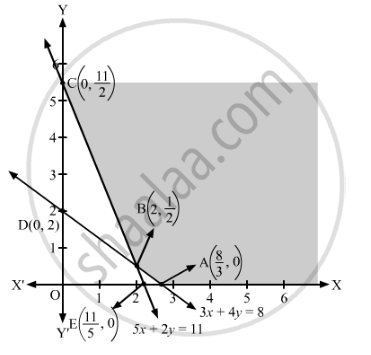# Reshma Wishes to Mix Two Types of Food P and Q in Such a Way that the Vitamin Contents of the Mixture Contains at Least 8 Units of Vitamin a and 11 Units of Vitamin B. - Mathematics

Sum

Reshma wishes to mix two types of food P and Q in such a way that the vitamin contents of the mixture contains at least 8 units of vitamin A and 11 units of vitamin B. Food P costs ₹60/kg and food Q costs ₹80/kg. Food P contains 3 units/kg of vitamin A and 5 units/kg of vitamin B while food Q contains 4 units/kg of vitamin A and 2 units/kg of vitamin B. Determine the minimum cost of the mixture.

#### Solution

Let x units of food P and units of food Q are mixed together to make the mixture.

The cost of food P is ₹60/kg and that of Q is ₹80/kg. So, x kg of food P and y kg of food Q will cost ₹(60x + 80y).

Since one kg of food P contains 3 units of vitamin A and one kg of food Q contains 4 units of vitamin A, therefore, x kg of food P and y kg of food Q will contain (3x + 4y) units of vitamin A. But, the mixture should contain atleast 8 units of vitamin A.

∴ 3x + 4≥ 8

Similarly, x kg of food P and y kg of food Q will contain (5x + 2y) units of vitamin B. But, the mixture should contain atleast 11 units of vitamin B.

∴ 5x + 2y  ≥ 11

Thus, the given linear programming problem is

Minimise Z = 60x + 80y

subject to the constraints

3x + 4≥ 8

5x + 2y  ≥ 11

x, y ≥ 0

The feasible region determined by the given constraints can be diagrammatically represented as,The coordinates of the corner points of the feasible region are A $\left( \frac{8}{3}, 0 \right)$ , B $\left( 2, \frac{1}{2} \right)$ and C $\left( 0, \frac{11}{2} \right)$

The value of the objective function at these points are given in the following table.

 Corner Point Z = 60x + 80y $\left( \frac{8}{3}, 0 \right)$ $60 \times \frac{8}{3} + 80 \times 0 = 160$ → Minimum $\left( 2, \frac{1}{2} \right)$ $60 \times 2 + 80 \times \frac{1}{2} = 160$ → Minimum $\left( 0, \frac{11}{2} \right)$ $60 \times 0 + 80 \times \frac{11}{2} = 440$

The smallest value of Z is 160 which is obtained at the points $\left( \frac{8}{3}, 0 \right)$  and  $\left( 2, \frac{1}{2} \right)$

It can be verified that the open half-plane represented by 60x + 80y < 160 has no common points with the feasible region.

So, the minimum value of Z is 160. Hence, the minimum cost of the mixture is ₹160.

Concept: Graphical Method of Solving Linear Programming Problems
Is there an error in this question or solution?

#### APPEARS IN

RD Sharma Class 12 Maths
Chapter 30 Linear programming
Exercise 30.3 | Q 10 | Page 40